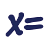Games
Problems
Go Pro!

# Consecutive Integers

Pro Problems > Math > Algebra > Equations > Word Problems > Linear > Consecutive Integers

# Featured Pro ProblemsThe sum of three consecutive integers is twenty-two more than twice the largest number. What are the three numbers?A simple algebra problem about two integers that are consecutiveConsecutive integer problem, involving an unknown number of integersFind the smallest of three consecutive integers, given information about their sumConsecutive integers whose sum is 31 less than three times the smallerSum of consecutive odd integer problem, single variable equationA single variable word problem involving consecutive odd integers

# Full Directory Listing

Consecutive Integers, Consecutive Odd Integers, Consecutive Sum, Consecutive Sum, Consecutives, Three Consecutive Integers, Three Consecutive IntegersLike us on Facebook to get updates about new resources